# Electro Thermal Modelling of Electrical Discharge Machining of Be-Cu Alloy by Varying Fraction of Energy

Electro Thermal Modelling of Electrical Discharge Machining of Be-Cu Alloy by Varying Fraction of Energy
S ‘O’A University, ITER, BBSR-751030, Odisha, India

Corresponding Author Email:
Pallavichoudhury@soa.ac.in
Page:
273-279
|
DOI:
https://doi.org/10.18280/acsm.430411
12 February 2019
|
Accepted:
20 May 2019
|
Published:
28 September 2019
| Citation

OPEN ACCESS

Abstract:

Beryllium Copper (Be-Cu) alloy are advanced engineering alloy bearing high strength, high wear resistance, Corrosion resistance and nonmagnetic nature. These properties of Beryllium copper make it impossible to machining through Conventional machining process. Electrical Discharge machining (EDM) is a revolution to machine any type of harder material (Electrically conductive) without any physical pressure. EDM is best suitable to machine Be-Cu alloy for small holes and intricate shape used in heat exchanger. To know the best suitable input parameters for improving the machining efficiency of EDM numerical analysis is always required. In this study a mathematical modeling of Be-Cu alloy machined by EDM has been performed by finite element modeling (FEM) method. A 3D axi-symmetric computational domain has been considered for the analyses confined by proper boundary condition. The numerical simulation has been performed for single discharge machining. The model has been validated with the experimental results. Simultaneously the Material Removal Rate (MRR) has been calculated with varying Discharge Current (I) (8, 10, 12 A), Fractions of heat (Fc) (18 %, 20 %, 22 %) and Pulse On time (Ton) (100,150, 200 μS). The lowest error of 4.55 % (in MRR) between numerical model and experimental results has been determined at 10A current.

Keywords:

finite element simulation, electrical discharge machining, material removal rate, plasma flushing efficiency

1. Introduction

Electric discharge machining (EDM) is an electro-thermal process where the electrical energy gets converted to highly energize thermal energy and that thermal energy helps to erode material from the work piece [1-3]. The metal erosion phenomena occur due to a series of electric spark which initiated between the electrode and the work piece, which leads to the breakdown of the dielectric liquid particles. This generate high amount of plasma in form of spark. In general, 50 % of total energy goes to the work piece which helps to erode material from it [2-3]. For the advancement of EDM mechanism process many advanced mechanisms like Ultrasonic assisted, Magnetic assisted, Wire EDM, Dry EDM, Rotary tool assisted, Powder mixed etc. mechanism have been evolved to get better output parameters like Material removal rate (MRR), Surface Roughness (SR), Overcut (OC) etc.

Many researchers since 1971 designed different types of analytical models for the theoretical analyses of EDM e.g., Dibitonto et al. ; Van Dijck and Dutre [10-15]; these models varied from each other by means of different boundary conditions. Kansal et al.  has been developed an axisymmetric 2D thermal model for AISID2 Die steel using the FEM. The model utilizes powder additives added in dielectric liquid along with other parameter to analyze the thermal profile and MRR for the EDM Process. The simulation shows that in this process smaller and shallower crater than normal EDM appears under the same set of machining conditions. The variation in prediction errors for MRR was found within ±5.5 %. As expected, the result shows that the maximum temperature is positioned at the core of workpiece, where intensity of heat flux (Gaussian heat distribution) is maximum and it decreases as move away from the centerline & it is represented by Eq. (8). Khazraji et al. [17-19] have been investigated a numerical analysis of EDM process by adding SiC powder mixed in EDM process using copper and graphite electrodes for machining ASTM-77 steel. The effect of powders has been investigated on the surface by white layer thickness (WLT). The reduced value of WLT of 5.0 μm and 5.57 μm has been reached for a high” current and low current with low pulse on time using SiC powder, respectively.

From the above Literature survey, it can be concluded that many researchers already performed the thermal modelling of EDM by taking various metals, alloys and composites as work piece [20, 21]. Many researchers have been studied the powder mixed EDM for improving the surface quality of work piece . But less work has been done for optimizing the best input parameters to get best output parameters . Less researcher has been performed the thermal modelling of Be-Cu alloy. The effect of various input parameters along with different fractions of heat entering in to the electrodes has been studied so far. The thermal modelling of Be-Cu alloy by considering the different fractions of heat has not been studied yet.

In this present study a thermal modelling has been performed for analysing the machining of Be-Cu alloy by EDM. Be-Cu is a highly electrical and thermal conductive alloy which is extensively used in heat exchangers like solar heat exchanger and welded heat exchanger [24, 25]. The Conventional mechanical technology is not suitable to machine these high degree précised components by Be-Cu alloy . Thermal analysis has been performed in this study to know the suitable range of input parameters to obtain a heist machining parameter by EDM. In this research the thermal modeling has been done by developing a 3D axisymmetric domain. The output parameters have been predicted with respect to different values of heat input or fractions of heat and has been validated with the experimental results.

2. Modeling Tethodology

In this study the FEM model for machining Be-Cu alloy by EDM has been introduced with the Experimental analysis. Although the fundamental principle of EDM is known, still it’s difficult to model the process due to the involvement of various mechanisms at cathode & anode electrode. There are mainly two types of boundary condition employed for the process firstly, heat due to Conduction secondly, heat transfer due to convection between the work piece & dielectric liquid. So the basic assumption for modeling the EDM process has been given below.

Assumption for Modeling:

1. The work piece has been considered as an axisymmetric domain.
2. The work piece material considered as homogeneous & isotropic.
3. The thermal properties of work piece are temperature independent.
4. Heat transfer due to radiation has been neglected.
5. Total work domain is considered to be 25 ℃.
6. Temperature effect along angular direction has been neglected.
7. Single spark discharge has been considered for the analyses.
8. Work piece considered to be free from residual stresses.

In Table 1 and Table 2 the basic thermo physical properties of Be-Cu alloy used in simulation has been presented.

Table 1. Mechanical & thermal properties of be-cu alloy

 Parameters Value/ Unit Density, ρ 8250 Kg/m3 Poisson's Ratio, γ 0.3 Tensile Yield Strength, σy 1030MPa Tensile Ultimate Strength, σUTS 1210MPa Melting Temptreature,Tm 865 ℃ Specific heat, Cp 0.1 Thermal Conductivity, K, (W/m2 ºC) 20 Co-efficient of thermal expansion,CTE(/˚C) 17*10-6

Table 2. Input parameters for methodology

 Parameters Value/ Unit Spark Radius. R 2.04*I0.44*Ton0.43 Dielectric medium Kerosene Film Coefficient, hf 1000 W/m2 ℃ Initial Temperature 25 ℃ Current, I 8, 10, 12 A Heat i/p to workpiece, Fc 5 % Pulse On time,Ton 100, 150,200µS Pulse Off time,Toff 50µS

2.1 Governing equation

In this study the simulation of EDM Process has been done by considering a 3D axisymmetric domain which is subjected to three different range of heat fraction entering in to cathode. The numerical solution has been obtained by solving the following Fourier heat conduction equation.

$\rho C_{\rho} \frac{\partial T}{\partial t}=\left[\frac{1}{r} \frac{\partial}{\partial r}\left(k \cdot \frac{\partial T}{\partial r}\right)+\frac{\partial}{\partial z}\left(k \frac{\partial T}{\partial z}\right)\right]$ (1)

where, ρ- Density of the work piece

Cp- specific heat of the work piece

K -Thermal conductivity of the wok piece

r, z are the coordinates of the axi-symmetrical work domain

T is the increase in temperature

2.2 Heat flux

From the literature review it has been cleared that the result obtained in the experimental EDM process has a close resemblance with the numerical model with Gaussian heat source. As the Gaussian heat flux is a function of spark radius over the time period so it has been considered as appropriate heat source for EDM machining.

$q=\frac{4.57 . F c J I}{\pi R^{2}} e^{-4.5\left(\frac{r}{R}\right)^{2}}$(2)

where, q - Total heat energy coming to the work piece

Fc -The fraction of heat entering in to the work piece

V- Voltage in V

I- Current (Amp)

In this study the determination of Radius of plasma channel has been based on Ikai and Hashiguchi . This Plasma radius is also known as “Equivalent Radius.

$R(t)=2.04 \times I^{0.43} \times T o n^{0.44}$ (3)

where, R is equivalent Spark Radius, I (A) is the Current and Ton(μS) is Pulse On time.

2.4 Boundary conditions

The schematic diagram of thermal model of EDM process for Be-Cu alloy has been represented in Figure 1. In this 3D axisymmetric domain has been converted to 2D domain. The Gaussian heat flux has been applied to the plasma channel/Spark radius (R). At boundary 4 convective boundary conditions has been applied due to the heat transfer to the dielectric liquid. As surface 2 & 5 are far from the conduction and convection area so they are subjected to thermal insulation. As the work piece is fully submerged inside the dielectric liquid throughout the process, so the initial temperature has been considered for the mathematical domain.

The initial boundary conditions are represented below in Eq.4:

Initial Condition at t=0, T (r, Z,0) =To

Boundary Condition for 3 (r<R)

$B C s: K\left(\frac{\partial T}{\partial z}\right)=\left\{\begin{array}{l}{h_{c}\left(T-T_{0}\right), r>R} \\ {q(r)(\text {Gaussian heat source}), r \leq R \text { on } B_{1}} \\ {0, \text { for off time}}\end{array}\right\}$ (4)

For boundary 1, 2, 5: = $\frac{\partial \cdot T}{\partial \cdot n}=0$

## 1.png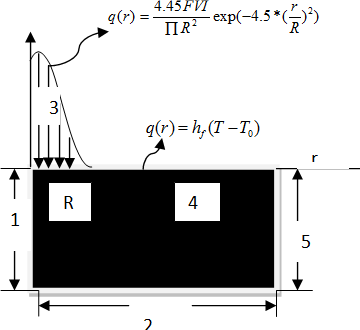Figure 1. Schematic boundary condition of the work domain

2.5 Modeling procedure

The numerical modeling has been done by COMSOL Multi-physics soft-ware. In COMSOL a 3D work domain has been designed for PMEDM analysis which has been subjected to transient heat transfer analysis. Figure 2 represents the 3D axisymmetric domain with meshing element. As already discussed the EDM process involves many complex mechanism so to simplify the modeling all the thermal properties of the work material are considered as temperature independent. All the input parameters have been taken according to tables 1 and 2. The meshed model contains 25 nodes with 12000 number of elements. These elements are hexahedral in shape. By solving Fourier heat conduction governing equation (equation-1) temperature profile has been obtained. From the temperature contour plot the selected region elements has been chosen (nodes which are grater then melting temperature). By eliminating those elements the required volume of the geometrical shape has been calculated which is used to determine the MRR. In table-3 three different sets of process parameters have been taken. Which are being used in modeling.

## 2.png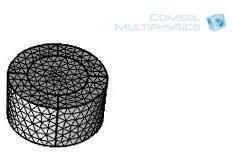Figure 2. Three dimensional view of meshed model

Table 3. Process variables setting

 Factors (Unit) Level-I Level-II Level-III Current (A) 8 10 12 Voltage (V) 30 40 50 Pulse On time (μS) 100 150 200 Fraction of heat i/p (Fc) 18 % 20 % 22 %
2.6 Determination of material removal rate

By applying the Gaussian heat flux the obtained crater shape can be considered as hemispherical bowl shape. Eqns. 5 and 6 represent the volume of crater for the hemispherical crater shape. The required shape has been obtained by eliminating the nodes (which are above the melting point of Be-Cu alloy i.e. 1145K) from the temperature profile obtained by solving the Fourier heat conduction equation has been presented in Figures 3 (a) and in (b) the hemispherical crater has been produced after eliminating the nodes which has greater melting point. Eq. 5 represents the volume of crater obtained for hemispherical bowl.

$C_{v}=\left\{\frac{1}{6} \pi h\left(3 r^{2}+h^{2}\right)\right\}$ (5)

## a.png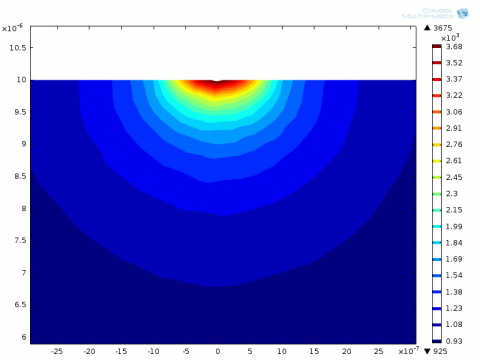(a)

## b.png(b)

Figure 3. (a) 2D Temperature Contour plot obtained after Simulation (b) Hemispherical Cavity shaped crater obtained after eliminating the elements with greater melting point

where, r - radius of spherical crater

h -depth of crater.

Tmach - machining time

Ton - Pulse-On time

Toff - Pulse-Off time.

In actual practice the MRR of the EDM Process depends on several factors like frequency of sparks, flushing efficiency, phase change of electrodes, random behaviour of debris particles, etc. Due to the complex mechanism of EDM it is critical to estimate the exact Thermal simulation. But by calculating the total crater volume Cv, the MRR (mm3/min) has been estimated by the following equation.

MRR= (Cv*NOP)/Tmach  (6)

where, Cv=Crater Volume (mm3)

NOP=Number of Pulse

Tmach=Machining time in minute

2.7 Validation with experiment

To strengthen the mathematical model the validation has been done by conducting the experiment with SMART ZNC Die sinking EDM machine. In general EDM oil has been used as dielectric, Be-Cu alloy as work piece and Cu-W as tool electrode. Figure 4 (a), (b), (c), (d) & (e) shows the experimental set up of EDM with Work piece before and after machining Condition respectively. The experiment has been conducted with reference to the process variable represent in Table 3. Table 4 represents the experimental results of Be-Cu alloy. All the experiment has been conducted by varying the current in three setting (by keeping other parameters as constant. From the table it can be concluded that minimum error has been calculated as 1.11 % at low current.

## abcde_.jpg(a)               (b)               (c)                  (d)

Figure 4. (a), (b), (c), (d) shows the experimental set up of EDM, Machining of Be-Cu alloy, Work piece before and after machining Condition respectively

Table 4. Experimental validation

 I (Amp) Ton (µs) Toff(µs) MRR (mm3/min) (EXP) R (µm) MRR (mm3/min) (FEM) PFE (%) Error in (%) 8 200 12 3.965 120 4.012 98.82 1.11 10 200 12 4.400 138.8 4.61 95.44 4.55 12 200 12 5.373 221.5 5.511 97.49 2.50
3. Results & Discussion

By solving equation-1 the temperature profile has been obtained from which the volume of crater (Cv) has been calculated by using Eq. 5. The erosion rate has been determined by using Eq. 6. To know the effect of different input parameters (Peak cur- rent, Voltage, Pulse-on time, Fraction of heat) on the machining of Be-Cu alloy the parametric study has been performed in the following section.

3.1 Effect of current

In Figure 5 the variation of the temperature along the spark radius at different Pulse On time has been plotted. The effect of different amount of current on temperature at Voltage-50V on work piece has been shown by the Figures 5, 6 & 7. In Figures 5, 6 & 7 all the increment of temperature line shows a bell shaped curve.

In Figure 5 the highest temperature recorded for 12A current is 4500K and in Figures 6 & 7 it is 8000k & 10000K at 12A current. From the above three figure it can be concluded that at high current the discharge gets in- creased due to which the surface temperature of the work piece gets increased. It can be shown from Figure 7 that at 8A the increment of temperature is around 5000K (Ton=200µS) which is similar to the trend line of Figure 6 at current 12A, which means the heat flux is directly proportional to the erosion rate process. All the curve shows a decrease rate towards the center of the radius and at 5E-5µ m the temperature gets lowered up to 273K. But at center of the work piece highest temperature recorded.

## 5.pngFigure 5. Effect of discharge current on the work piece surface at Ton=100 µS

## 6.pngFigure 6. Effect of discharge current on the work piece surface at Ton=150 µS

## 7.pngFigure 7. Effect of discharge current on the work piece surface at ton=200 µS

3.2 Effect of pulse duration

In Figures 8, 9 the temperature variation on the surface of the work piece (along the radius & depth) has been shown at volt- age -70V for three different Pulse On time. When the thermal energy in form of heat flux supplied for a longer period of time the temperature increase on the work piece surface. It decreases slowly as we move away from the workpiece. For example At Ton 100µS(I=10A) the temperature increases up to 2000K, Similarly at Ton 150µS (I=10A) the temperature raises up to 8000K. Similarly at deeper radial penetration the temperature decreases. This is due to the temperature gradient of longer pulse.

## 8.png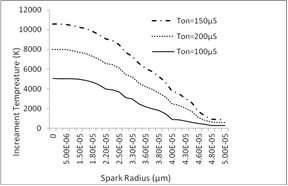Figure 8. Effect of pulse on time on the work piece surface along at i=10 A

## 9.png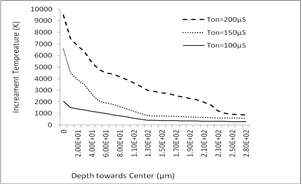Figure 9. Effect of pulse on time on the work piece at radial depth from the center at i=10 A

3.3 Effect of fraction of heat

From Eq. 2 it has been cleared that the total thermal energy or heat flux directly proportional to the fraction of heat entering in to the work piece or cathode. In this study three different fraction of heat i.e. 0.18, 0.2, 0.22 has been taken for the modeling of Be-Cu alloy machining by EDM process. From figure-10 it has been cleared that at Fc-0.22 the temperature rises around 8000K. Similarly, at different i-10A the highest temperature recorded at Fc-0.22. As current and Fc directly proportional to the amount of heat energy so the temperature increases due to increase in current and Fc.

Figure 13 (a), (b) & (c) shows the temperature contour of the work domain after single discharge simulation at i-10A, V-30V and Ton- 100µS. From the figure it can be cleared that the crater obtained from the application of Gaussian heat flux looks like a hemispherical bowl shape. This figure is helpful to estimate the volume of crater and material removal rate.

## 10.png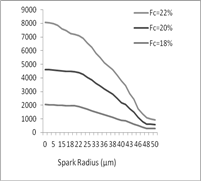Figure 10. Effect of Fc along the radial direction at i=8A(Ton=100µS)

## 11.pngFigure 11. Effect of Fc along the radial direction at i=10A (Ton=100µS)

## 12.pngFigure 12. Effect of Fc along the radial direction at i=12A (Ton=100µS)

## aaaaa.png(a)

## bb.png(b)

## cccccc.png(c)

Figure 13. (a), (b), (c)Thermal profile of cut plane for varying Fc=0.18, 0.20, 0.22 at i=10A, V=30V & Ton=100µS

4. Conclusion

In this present research the effect of fraction of energy to the work electrode on machining of Be-Cu alloy by powder mixed EDM has been investigated. For this study a Finite element modelling (FEM) has been developed by designing a 3D axisymmetric computational domain. The FEM results has been verified with the experimental results.

Ø The predicted result by applying Gaussian heat source shows a good agreement with the experimental results of EDM.

Ø The Material removal rate is directly proportional to the supplied current, voltage and pulse-on time.

Ø Among the various fractions of heat the 20% Fc shows the least deviation i.e 4.55% from the experimental results.

Ø The highest crater radius obtained at high current is 4E-5 μm similarly at high Pulse duration the highest crater radius is 5E-5 μm.

Ø The highest crater depth obtained at high current and high spark –on time is 2.8E-2 μm.

Ø The temperature growth curve for crater radius follows a bell shaped curve.

Ø The temperature isotherm obtained after single discharge simulation looks like a hemispherical bowl.

The removal efficiency by EDM increases by increasing the thermal energy by considering the maximum 20 % of heat fraction entering in to the work piece surface.

5.Acknowledgment

Lastly, we would like to thanks ITER Mechanical Engineering Department HOD & ADVANCED MACHINING LABORATORY assistant who helped a lot for conducting the experiments by EDM.

References

 Zeid, O.A.A. (1997). On the effect of electro-discharge machining parameters on the fatigue life of AISI D6 tool steel. Journal of Materials Processing Technology, 68(1): 27-32. https://doi.org/10.1016/S0924-0136(96)02523-X

 Koshy, P., Jain, V.K., Lal, G.K. (1993). Experimental Investigations into electrical discharge machining with a rotating disc electrode. Precision Engineering, 15(1): 6-15. https://doi.org/10.1016/0141-6359(93)90273-D

 Soni, J.S., Chakraverti, G. (1994). Machining characteristics of Titanium with rotary electro-discharge machining. Wear, 171(1-2): 51-58. https://doi.org/10.1016/0043-1648(94)90347-6

 Yan, B.H., Wang, C.C., Chow, H.M., Lin, Y.C. (2000). Feasibility study of rotary electrical discharge machining with ball burnishing for Al2O3/6061Al composite. International Journal of Machine Tools and Manufacture, 40(10): 1403-1421. https://doi.org/10.1016/S0890-6955(00)00005-5

 Zhao, W.S., Meng, Q.G., Wang, Z.L. (2002). The application of research on powder mixed EDM in rough machining. Journal of Materials Processing Technology, 129: 30-33. https://doi.org/10.1016/S0924-0136(02)00570-8

 Tzeng, Y.F., Lee, C.Y. (2001). Effects of powder characteristics on electro discharge machining efficiency. International Journal of Advanced Manufacturing Technology, 17: 586-592. https://doi.org/10.1007/s001700170142

 Mohri, N., Saito, N., Higashi, M. (1991). A new process of finish machining on free surface by EDM methods. Annals of CIRP, 40(1): 207-210. https://doi.org/10.1016/S0007-8506(07)61969-6

 Erden, A., Bilgin, S. (1980). Role of impurities in electric discharge machining, in: Proc. 21st Int. Machine Tool Design and Re- search Conference, Macmillan, London, pp. 345-350. https://doi.org/10.1007/978-1-349-05861-7_45

 Ming, Q.Y., He, L.Y. (1995). Powder-suspension dielectric fluid for EDM. Journal of Material Processing Technology, 52: 44-54. https://doi.org/10.1016/0924-0136(94)01442-4

 DiBitonto, D.D., Patel, M.R., Barrufet, M.A., Eubank, P.T. (1989). Theoretical models of the electrical discharge machining process. I. A simple cathode erosion model. Journal of Applied Physics, 66(9): 4095-4103. https://doi.org/10.1063/1.343994

 Dijck, F.V. Dutre, W. (1974). Heat conduction model for the calculation of the volume of molten metal in electric discharges. Journal of Physics D: Applied Physica, 7(6): 899-899. https://doi.org/0022-3727/7/6/316

 Snoeys, R., Staelens, F., Dekeyser, W. (1986). Current trends in non-conventional material removal processes. CIRP Annals, 35(2): 467-480. https://doi.org/10.1016/S0007-8506(07)60195-4

 Beck, J.V. (1981). Transient temperatures in a semi-infinite cylinder heated by a disk heat source. International Journal of Heat & Mass Transfer, 24(10): 1631-1640. https://doi.org/10.1016/0017-9310(81)90071-5

 Jilani, S.T., Pandey, P. (1982). Analysis and modeling of EDM parameters. Precisions Engineering, 4(4): 215-221. https://doi.org/10.1016/0141-6359(82)90011-3

 Joshi, S., Pande, S. (2010). Thermo-physical modelling of die-sinking EDM process. Journal of Manufacturing Process, 12(1): 45-56. https://doi.org/10.1016/j.jmapro.2010.02.001

 Salonitis, K. (2009). Thermal modeling of the material removal rate and surface roughness for die-sinking EDM. International Journal of Advance Manufacturing Technology, 40(3-4): 316-323. https://doi.org/10.1007/s00170-007-1327-y

 Kansal, H.K., Singh, S., Kumar, P. (2008). Numerical simulation of powder mixed electric discharge machining (EDM) using finite element method. Mathematical and Computer Modelling, 47(2008): 1217-1237. https://doi.org/10.1016/j.mcm.2007.05.016

 Al-Khazraji, A., Amin, S.A., Ali, M.S. (2016). The effect of Graphite Flakes powder mixing electrical discharge machining on white layer thickness, heat flux and fatigue life of AISI D2 die steel. Engineering Science and Technology, an International Journal. 19(3): 1400-1415. https://doi.org/10.1016/j.jestch.2016.01.014

 Tan, P.C., Yeo, S.H. (2013). Simulation of surface integrity for nanopowder-mixed dielectric in micro electrical discharge machining. Metallurgical & Material Transactions B, 44B. https://doi.org/10.1007/s11663-013-9819-7

 Chaudhury, P., Samantary, S. (2019). Finite element modelling of EDM of aluminum particulate metal matrix composites considering temperature dependent properties. Revue des Composites et des Materiaux Avances, 29(1): 53-62. https://doi.org/10.18280/rcma.290109

 Chaudhury, P., Samantaray, S., Sahu, S. (2017). Multi response optimization of powder additive mixed electrical discharge machining by Taguchi analysis. Mater Today Proceeding, 4: 2231-2241.

 Chaudhury, P., Samantaray, S., Sahu, S. (2017). Optimization of process parameters of powder additive-mixed electrical discharge machining. In: Innovative Design and Development Practices in Aerospace and Automotive Engineering, ed: Springer, pp. 415-425.

 Chaudhury, P., Samantaray, S. (2017). Role of carbon Nano Tubes in surface modification on electrical discharge machining -a review. Mater Today: Proceeding, 4(2, Part A): 4079-4088.

 Lindero-Hernández, M., Ramos-López, G., Nieto-Pérez, M. (2019). Evaluation of copper–beryllium contacts for high current density pulsed applications. In IEEE Transactions on Plasma Science, 47(8): 4048-4051. https://doi.org/10.1109/TPS.2019.2925382

 Lee, D. (2018). Experimental investigation of laser ablation characteristics on nickel-coated beryllium copper. Metals, 8(4): 211. https://doi.org/10.3390/met8040211